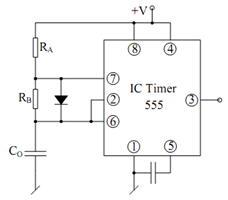## Expression for the time period Assignment Help

Assignment Help: >> Basic Astable Multivibrator - Expression for the time period

Expression for the time period:

An expression for the time period of the output waveform can be determined as follows. The equation for the capacitor charging may be written in the following general form.

Vc (t ) = A + B e - t /( RA + RB ) C

where A and B are constants which may be resolute from the following conditions.

at t = 0,              Vc (t ) =      Vcc / 3

at t = ∞ ,         Vc (t) = Vcc

Substituting in Eq. (97), we determine

Vcc  = A + B and Vcc  = A

from which it is found that

∴          B = 2Vcc /3

Eq. (97) now becomes

V (t) = Vcc  - (2/3) Vcc e- t /( RA + RB ) C

Now at t  = TH, VC (t) =(2/3)Vcc ,therefore, we get

(2 /3)V cc        = V cc  - (2/3) V cc   e (- tH/( RA + RB ) C)

(2/3) e - tH/ ( RA + RB ) C  = 1/3

e tH/ ( RA + RB ) C  = 2

from which the needed time period is given by

TH  = (RA  + RB ) C ln 2

likewise, one can determine that considering the equation for discharge of the capacitor with time constant CRB, one may find TL as

TL  = RB C ln 2

The frequency of the output waveform is, thus, given by

f  =       1 / (RA  + RB ) C ln 2

and the duty cycle is given by

f  =  (RA  + RB ) (RA  + 2RB )

Note down that even if one selects RA = RB, the duty cycle may not be made 50%. However, the duty cycle may be made 50% by RB shunting by a diode as illustrated in FigureFigure: Astable Multivibrator with 50% Duty Cycle

This makes TH = RA C ln 2 and TL= RB C ln 2, so that the duty cycle is given by

δ=       RA /RA  + RB

which would become 50% for RA = RB.